SELF 14 S.B. Karavashkin and O.N. Karavashkina6. Relativistic way to general covariance through the violation of conservation laws Speaking of especial mathematical way of relativistic cognition, we cannot avoid the issue of term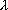, which Einstein introduced to his equations of GR to achieve the general covariance. To understand this problem, we have to answer a very simple question: where from this problem appeared? In frames of classical formalism it not only never arose, it was absent as such. As J. Norton  correctly points, the problem arose when Einstein "came to conclusion that the equivalency principle which he advanced gives the clue to the relativistic theory of gravitation and to generalisation of relativity principle" [32, p. 57]. Later Einstein wrote on this issue the following: "Considerable achievement of general theory of relativity is, it saved the physics from the necessity to introduce the 'inertial frame' (either 'inertial frames'). This concept is not satisfactory, because it without any grounds (? - Authors) selects some systems from all ever minded coordinate systems. Then it is premised that the laws of physics are true only for such inertial systems (e.g., the law of inertia and the law of constant speed of light) (? - Authors). Thus, in the system of physics, the space as such is endowed with the role that selects it among all other elements of physical description. It takes a definitive part in all processes, being not affected by a feedback (? - Authors). Although such theory is logically possible, it seems to be not fully satisfying" [33, p. 854]. As we will see below, such neglect of the basic laws of nature discovered by Newton automatically caused a whole link of violations which Einstein had to admit, having unfoundedly rejected the inertial reference frame, and this rejection originated from the very beginning of his work on the project of Relativity. "The crucial results were obtained in 1912 and 1913, after Einstein arrived back to Zurich. Here Einstein founded the mathematical basis of general theory of relativity, using in mathematical issues the help of his friend Marcel Grossmann. But the theory which they built … had a defect, as the authors did not succeed to involve to it the generally covariant equations of gravity field" [32, p. 57]. The cause was same. Einstein, having addressed to the generalisation of gravity laws, directed these efforts not to deepen the understanding of phenomenology of gravity field but to guess such geometric metric which by its outward parameters would seem like a motion of particle in the gravity field. As Norton evidences, in his Zurich notebook, "up to 3 006 14L, Einstein unsuccessfully runs over different simple expressions in a hope to find a suitable tensor; in the mentioned page he for first time explicitly writes down the Riemann curvature tensor. Most probably, it was Grossmann who told him the expression for the curvature tensor, since it is written near it: 'tensor of fourth rank by Grossmann' " [32, p. 62]. The features of such way of 'development' of physical ideas has been naturally reflected in the basic work by Einstein together with Grossmann "The project of generalised theory of relativity and theory of gravitation": "In the generalised vector analysis, the Christoffel four-sign symbols of second kind have a sense of components of mixed tensor contravariant in three signs and covariant in one sign. The main importance of these concepts for differential geometry of diversity given by its linear element (identical equality to zero of tensor Rikm is the necessary and sufficient condition in order the differential form could be reduced to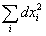- Authors) allows us to premise a priori that these generalised differential tensors can appear useful also in composing the differential equations of gravity field. Actually, we can point at once the covariant tensor of the second rank and second order Gim , which could be involved in these equations, namely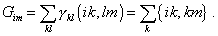(57)
 But in particular case of infinitely weak static field of gravity, this tensor is unreducable to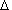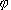. So the question remains open, how much extended is the relation of the problem of gravity field equations in general theory of differential tensors connected with gravity field" [34, p. 262]. But factually Einstein understood, the difficulty was that to the physical part of project the conservation laws were involved as Poisson equations: "Let the tensor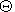to be given for a material system. Which will be the differential equations allowing to determine the values gik , i.e. the gravity field? In other words, we are seeking the generalisation of Poisson equation,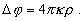(58)
 To solve this problem, we could not find a method which would be so natural as in case of previous problem (seeking the expression for the tensor of energy-momentum - Authors), We had to introduce some far from being obvious, though probable admissions. The sought equation, most probably (italicised by us - Authors) has to be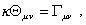(59)
 whereis the constant,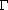is the contravariant tensor of the second rank formed from the basic tensor g" [34, p. 236]. It would seem, a clear discrepancy in the methodology of Einstein's approach and reality would have to cause him to correct the methodology, but only in case if Einstein did not profess Relativity. In accordance with it, in case of discrepancy in relativistic conception and reality, it is reality what they think to be incorrect. So immediately after Einstein introduced (59), he unfoundedly doubts the Poisson conservation law and Newton law: "Accordingly to the law of Newton - Poisson, it seems sensible to require, the equations (59) to be the equations of the second order. But we have to object, this premise disables us to find the differential equation being the generalisation ofwhich would be the tensor relatively the arbitrary transformations" [34, p. 236- 237]. In other words, if they cannot write down the conservation law in the 4 D form of Einstein - Minkowski, the conservation law is invalid. And even more, "we cannot state a priori that final exact equations of gravity cannot contain the derivatives of order higher than second. So there still exists the possibility that final exact differential equations of gravity can be covariant as to the arbitrary transformations. But with today state of our knowledge of physical properties of gravity field, the discussion on such possibilities would be beforehand. So we have to confine ourselves to the second-order equations and, hence, to give up the search of gravity equations covariant as to the arbitrary transformations. It is necessary, however, to underline that we have no grounds for general covariance of gravity equations" [34, p. 237]. From this citation we clearly see that Einstein not for an instant was falling to thinking, whether his wishes are consistent with reality. He is ready to put off any proof, any conservation law, nothing to say of natural regularities, striving to state his geometrical formalism true. And contradictions that arose in his work are the direct consequence of neglecting the basic principles of methodology of cognition that have been formed in classical physics. Well, what in essence intended Einstein to fulfil? "With the help of absolute differential calculus developed by Ricci and Levi-Civita, on the basis of mathematical study by Christoffel, we succeed (? - Authors) to substitute the known systems of equations of theoretical physics for such equivalent (in case of constant g) equations that would be absolutely true, irrespectively of the choice of space-time coordinates x. All such systems of equations contain the values g, i.e. the values, determining the gravity field. So this last affects all physical processes" [35, p. 393]. In other words, in his project Einstein intended to elucidate the equations of gravity from the equations of motion, introducing the gravity forces as some parameters warping the metric; later Reichenbucher pointed him, this is inadmissible. Einstein answered in the favourite relativistic style: "The example of gravity fields vanishing in coordinate transformation is important only as a known particular case in which the sought laws of nature are known to be true" [36, p. 691]. None the less, the difficulty retained. Its meaning was, in classical physics we also can pass to a coordinate system related to the body freely falling in the gravity field, but this reference frame is non-inertial and in it, naturally, the conservation laws are not fully valid. While Einstein in his "Project…"  tried to retain the linear metric in transition to nonlinear reference frame. Another discrepancy has 'sedimented' on this. It was connected with the impossibility to describe in the 4D appearance the stationary gravity fields. This required from Einstein to introduce a far from being obvious principle of equivalence, which only outwardly was formulated as the principle of equivalence of inertial and weighty masses. Should the meaning of this principle were namely such, Newton long before Einstein established it, having introduced the relationship between the force affecting the body in the field with the gravity force, as follows: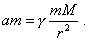(60)
 We see from (60) that in its left part the mass m is involved as the measure of body's inertia determining its acceleration under affection of the force, and in the right part the mass m has been written as the weighting and providing the gravity interaction. But Einstein's task was to identify the laws in the inertial and non-inertial reference frames, reducing the body's motion in the gravity field to an usual accelerated motion. "An adherent of trivial modern theory of relativity with a definite degree of rightness calls the speed of material point 'seeming'. Namely, he can choose the reference frame so that the material point to have at the considered moment the zero speed. But if there is the system of material points that have different speeds, it already cannot introduce such reference frame relatively to which the speeds of all points will turn to zero. Similarly a physicists who stands for our view can call 'seeming' the gravity field, as through a definite choice of reference frame acceleration he can achieve, at a definite point of space-time the gravity field to turn to zero. But it is noteworthy that turning the gravity field to zero through the transformation is unachievable in general case for extensive gravity fields. For example, we cannot make zero the Earth's gravity field through choosing a suitable reference frame" [37, p. 285]. To the said Einstein adds an important stipulation: "If to describe the nature it might appear necessary to use an arbitrarily chosen coordinate system, the choice of the system has to be unlimited; physical laws have to be fully independent of this choice (general principle of relativity)" [38, p. 679]. And this really was Einstein to whom we have to attribute the identifying of the laws of nature in inertial and non-inertial reference frames.

Contents: / 1 / 2 / 3 / 4 / 5 / 6 / 7 / 8 / 9 / 10 / 11 / 12 / 13 / 14 / 15 / 16 / 17 /